# Coulombs law Objectives Describe the historical development of

• Slides: 40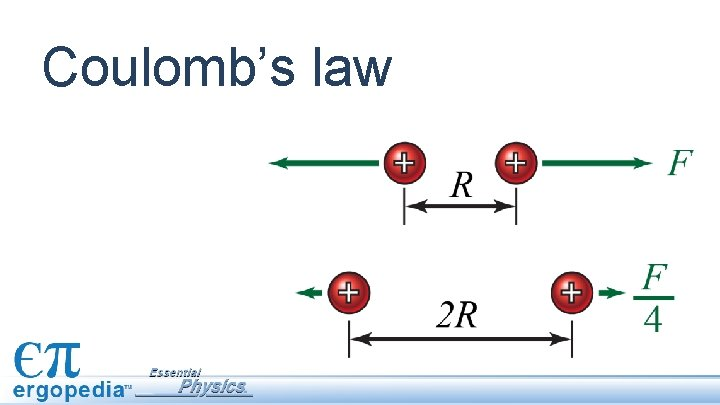Coulomb’s law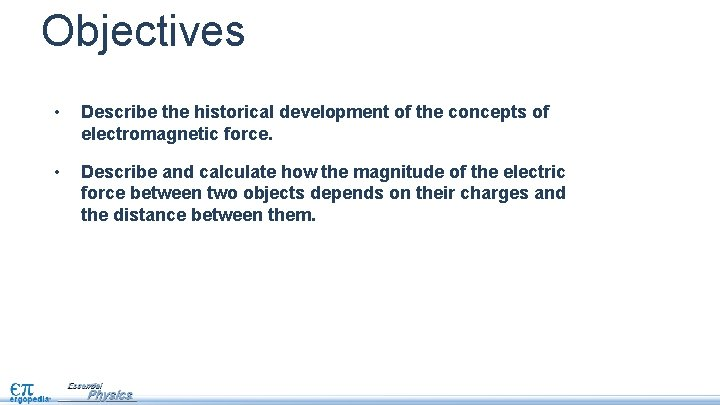Objectives • Describe the historical development of the concepts of electromagnetic force. • Describe and calculate how the magnitude of the electric force between two objects depends on their charges and the distance between them.Assessment 1. For whom was the unit of charge, the coulomb, named and in what way(s) did this person contribute to our understanding of the electric force? 2. Two charged particles are located 1. 0 m apart. Describe the direction for the electric force between them if the two charges are: a. +1 μC and +1 μC b. +1 μC and -1 μC c. -1 μC and -1 μCAssessment 3. Two charged particles are located 1. 0 m apart. a) Calculate the magnitude of the electric force between them if the two charges are +1. 0 μC and +1. 0 μC. a) What would be the magnitude of the force if the charges were 50 centimeters apart?Physics terms • Coulomb’s law • coulombEquations The force between point charges is proportional to the product of the charges, divided by the square of the distance between them.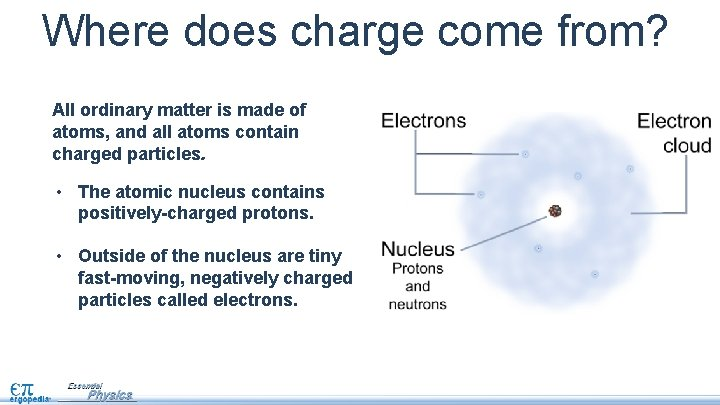Where does charge come from? All ordinary matter is made of atoms, and all atoms contain charged particles. • The atomic nucleus contains positively-charged protons. • Outside of the nucleus are tiny fast-moving, negatively charged particles called electrons.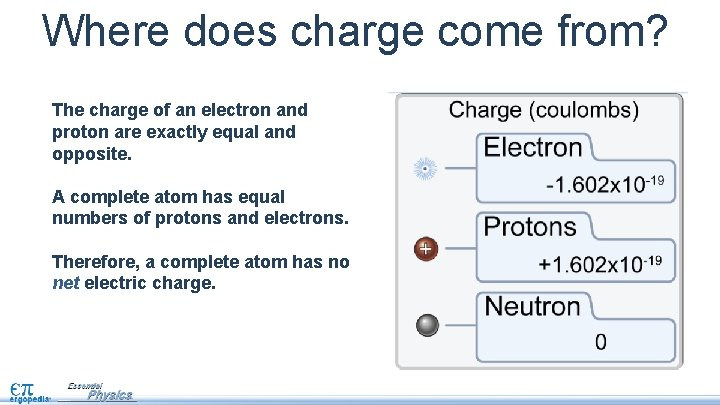Where does charge come from? The charge of an electron and proton are exactly equal and opposite. A complete atom has equal numbers of protons and electrons. Therefore, a complete atom has no net electric charge.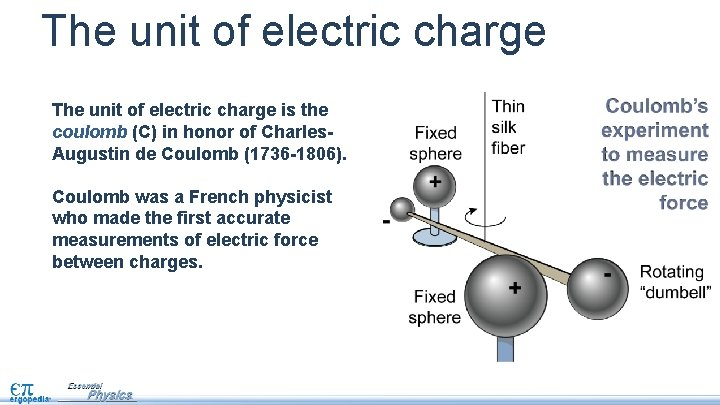The unit of electric charge is the coulomb (C) in honor of Charles. Augustin de Coulomb (1736 -1806). Coulomb was a French physicist who made the first accurate measurements of electric force between charges.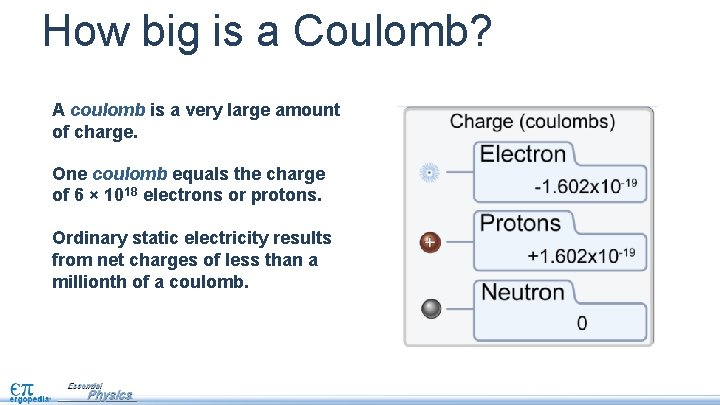How big is a Coulomb? A coulomb is a very large amount of charge. One coulomb equals the charge of 6 × 1018 electrons or protons. Ordinary static electricity results from net charges of less than a millionth of a coulomb.Think: How can you increase the repulsive force between two positive charges? What does this force depend on? Fe Fe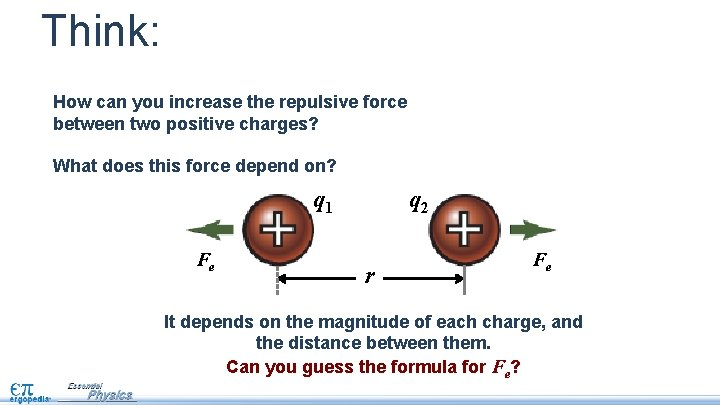Think: How can you increase the repulsive force between two positive charges? What does this force depend on? q 1 Fe q 2 r Fe It depends on the magnitude of each charge, and the distance between them. Can you guess the formula for Fe?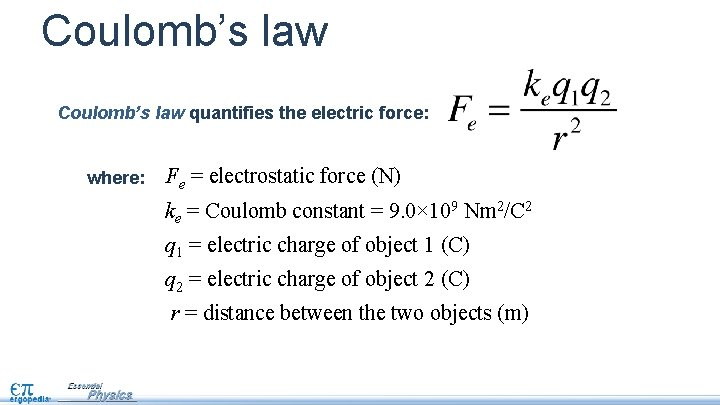Coulomb’s law quantifies the electric force: where: Fe = electrostatic force (N) ke = Coulomb constant = 9. 0× 109 Nm 2/C 2 q 1 = electric charge of object 1 (C) q 2 = electric charge of object 2 (C) r = distance between the two objects (m)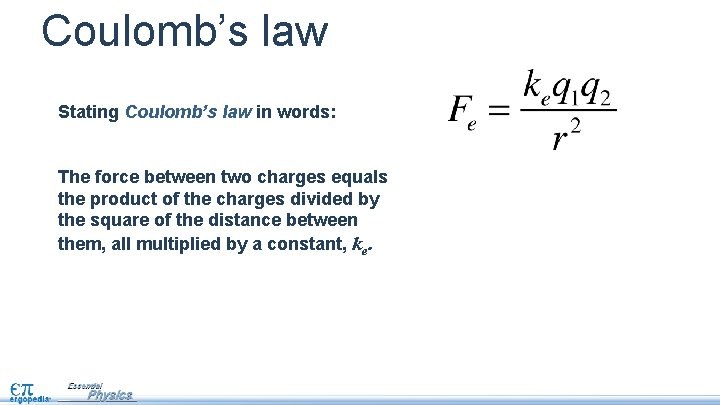Coulomb’s law Stating Coulomb’s law in words: The force between two charges equals the product of the charges divided by the square of the distance between them, all multiplied by a constant, ke.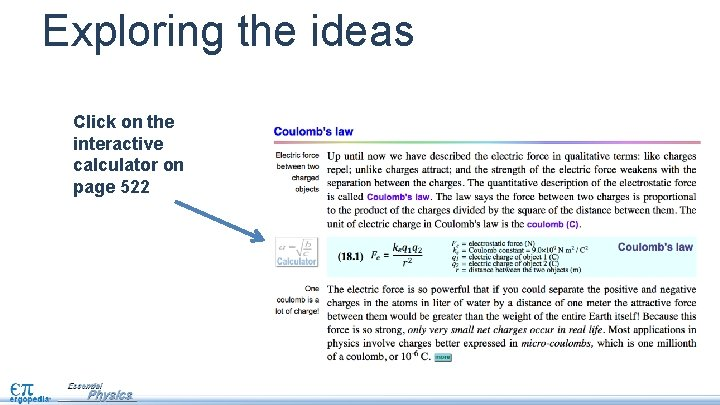Exploring the ideas Click on the interactive calculator on page 522Engaging with the concepts Two silver balls with charges of +7. 0 μC and +1. 2 m. C, are located 80 cm apart. Electric force What is the force between them? 7 e-6 7. 0 e-6 1. 2 e-3 1. 2 e-3 0. 80 8. 99 e+9 0. 80 In what direction does it act? Hint: make sure you use the right units!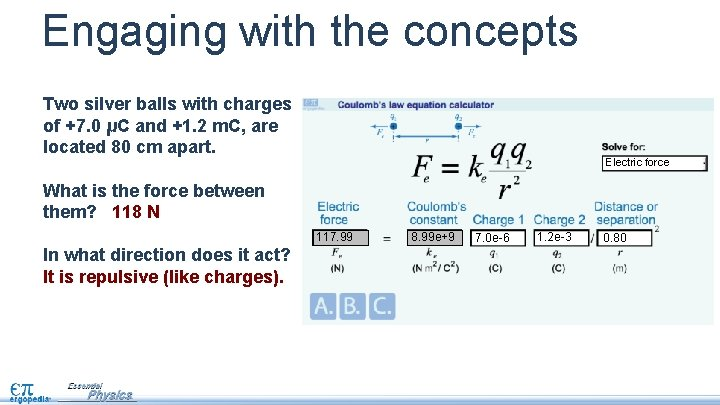Engaging with the concepts Two silver balls with charges of +7. 0 μC and +1. 2 m. C, are located 80 cm apart. Electric force What is the force between them? 118 N 117. 99 In what direction does it act? It is repulsive (like charges). 7 e-6 7. 0 e-6 1. 2 e-3 1. 2 e-3 0. 80 8. 99 e+9 0. 80Engaging with the concepts Two small metal spheres are separated by 25 mm. One has a charge of +2. 0 μC, the other − 2. 0 μC. What is the force between them? Electric force 7 e-6 2. 0 e-6 1. 2 e-3 -2. 0 e-6 0. 80 8. 99 e+9 0. 025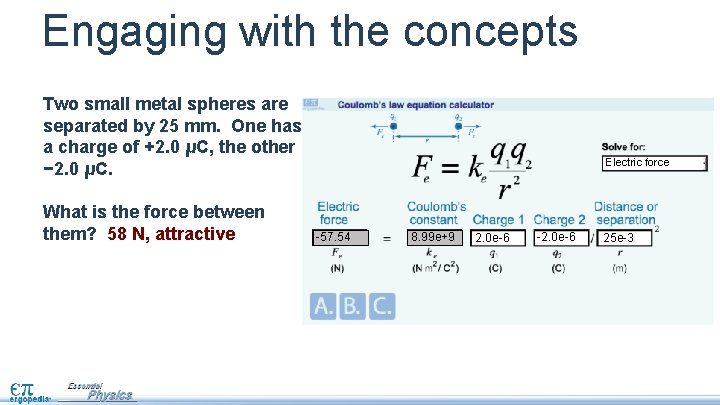Engaging with the concepts Two small metal spheres are separated by 25 mm. One has a charge of +2. 0 μC, the other − 2. 0 μC. What is the force between them? 58 N, attractive Electric force -57. 54 7 e-6 2. 0 e-6 1. 2 e-3 -2. 0 e-6 0. 80 8. 99 e+9 25 e-3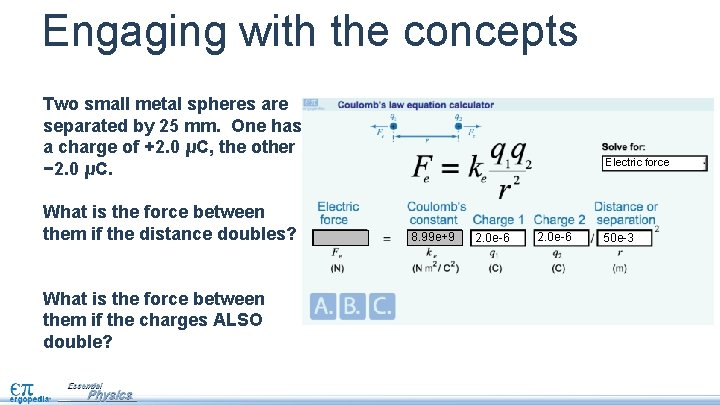Engaging with the concepts Two small metal spheres are separated by 25 mm. One has a charge of +2. 0 μC, the other − 2. 0 μC. What is the force between them if the distance doubles? What is the force between them if the charges ALSO double? Electric force 7 e-6 2. 0 e-6 1. 2 e-3 2. 0 e-6 0. 80 8. 99 e+9 50 e-3Engaging with the concepts Two small metal spheres are separated by 25 mm. One has a charge of +2. 0 μC, the other − 2. 0 μC. What is the force between them if the distance doubles? 1/4 as much: 14 N What is the force between them if the charges ALSO double? still 58 N Electric force -14. 38 7 e-6 2. 0 e-6 1. 2 e-3 2. 0 e-6 0. 80 8. 99 e+9 50 e-3Fe increases with charge The electric force increases with increasing charge:Fe increases with charge The electric force increases with increasing charge: Doubling one charge doubles the force:Fe increases with charge The electric force increases with increasing charge: Doubling one charge doubles the force: Doubling both charges quadruples the force: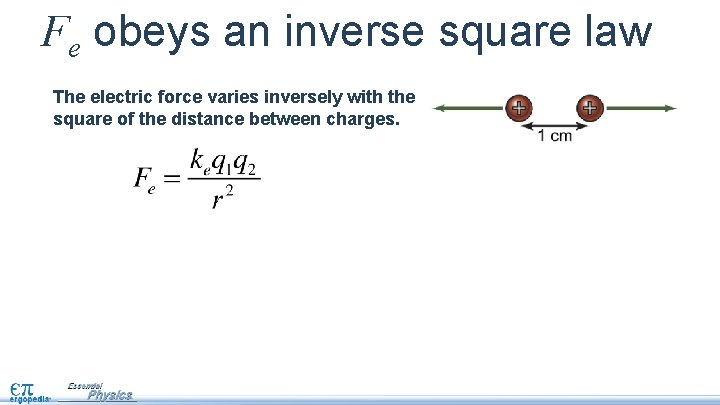Fe obeys an inverse square law The electric force varies inversely with the square of the distance between charges.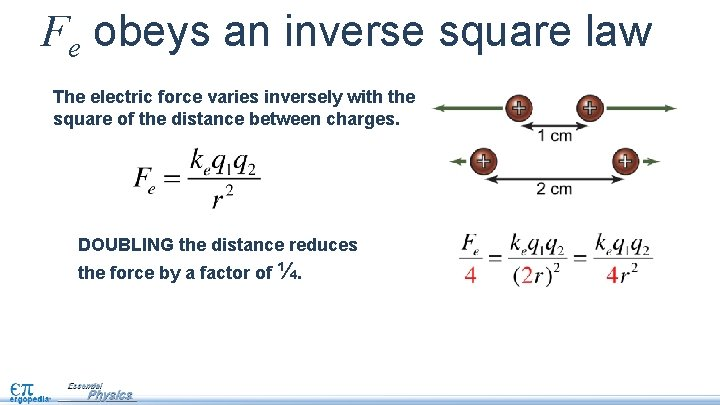Fe obeys an inverse square law The electric force varies inversely with the square of the distance between charges. DOUBLING the distance reduces the force by a factor of ¼.Fe obeys an inverse square law The electric force varies inversely with the square of the distance between charges. DOUBLING the distance reduces the force by a factor of ¼. HALVING the distance increases the force by a factor of 4.Test your knowledge Two +1. 0 μC charges are separated by a distance of 1. 0 cm. What is the magnitude and direction of their mutual electric force? Asked: Given: r Relationships: Solution:Test your knowledge Two +1. 0 μC charges are separated by a distance of 1. 0 cm. What is the magnitude and direction of their mutual electric force? Asked: force Given: charge q 1 and q 2, distance r Relationships: Solution: The force is repulsive.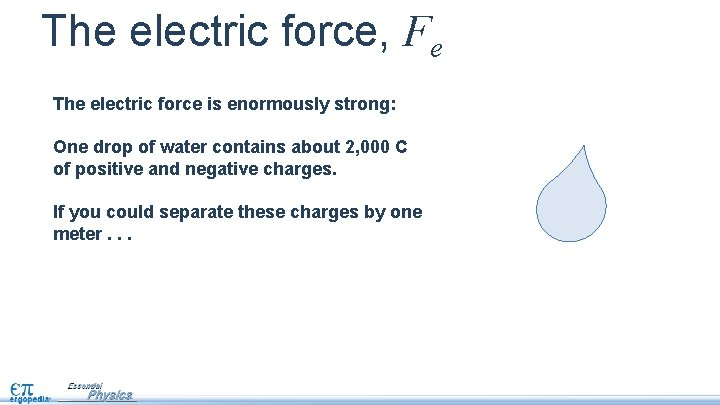The electric force, Fe The electric force is enormously strong: One drop of water contains about 2, 000 C of positive and negative charges. If you could separate these charges by one meter. . .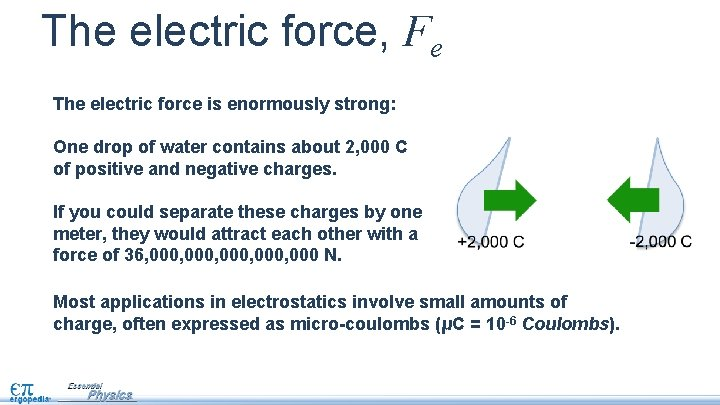The electric force, Fe The electric force is enormously strong: One drop of water contains about 2, 000 C of positive and negative charges. If you could separate these charges by one meter, they would attract each other with a force of 36, 000, 000 N. Most applications in electrostatics involve small amounts of charge, often expressed as micro-coulombs (μC = 10 -6 Coulombs).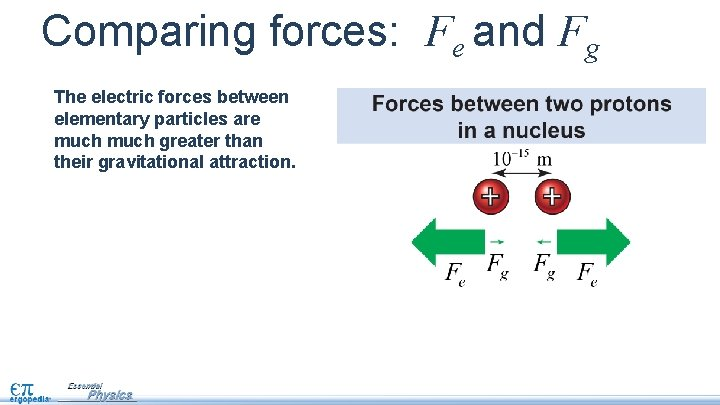Comparing forces: Fe and Fg The electric forces between elementary particles are much greater than their gravitational attraction.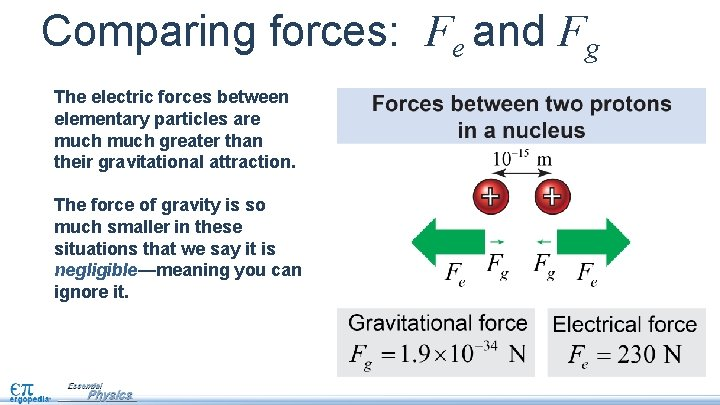Comparing forces: Fe and Fg The electric forces between elementary particles are much greater than their gravitational attraction. The force of gravity is so much smaller in these situations that we say it is negligible—meaning you can ignore it.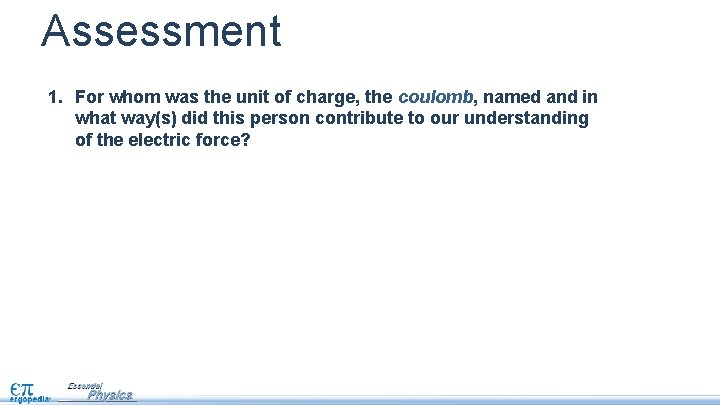Assessment 1. For whom was the unit of charge, the coulomb, named and in what way(s) did this person contribute to our understanding of the electric force?Assessment 1. For whom was the unit of charge, the coulomb, named and in what way(s) did this person contribute to our understanding of the electric force? answer: Charles-Augustin de Coulomb (1736 -1806) was a French physicist who made the first accurate measurements of the electrical force between charges.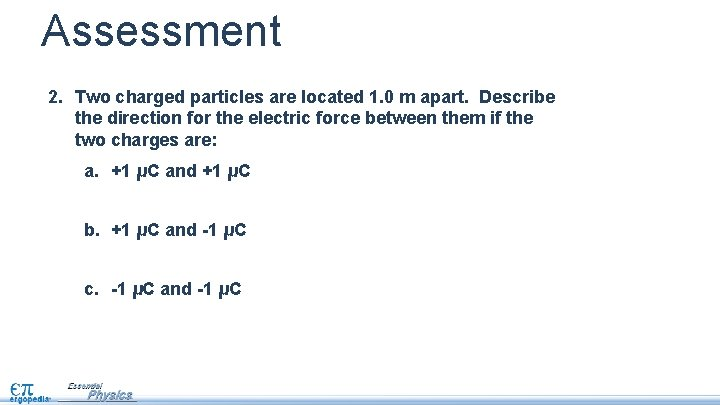Assessment 2. Two charged particles are located 1. 0 m apart. Describe the direction for the electric force between them if the two charges are: a. +1 μC and +1 μC b. +1 μC and -1 μC c. -1 μC and -1 μCAssessment 2. Two charged particles are located 1. 0 m apart. Describe the direction for the electric force between them if the two charges are: a. +1 μC and +1 μC repulsive b. +1 μC and -1 μC attractive c. -1 μC and -1 μC repulsive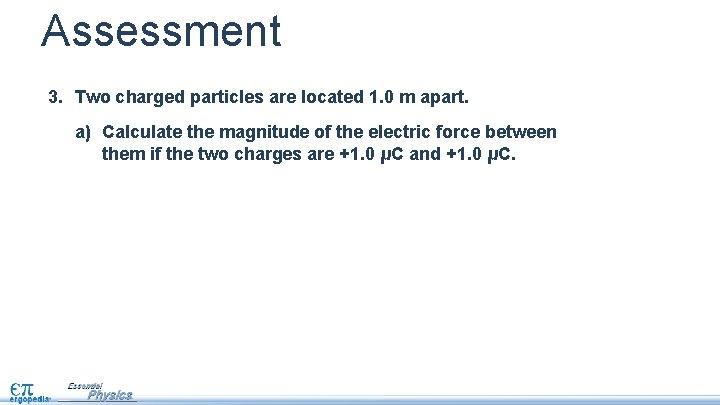Assessment 3. Two charged particles are located 1. 0 m apart. a) Calculate the magnitude of the electric force between them if the two charges are +1. 0 μC and +1. 0 μC.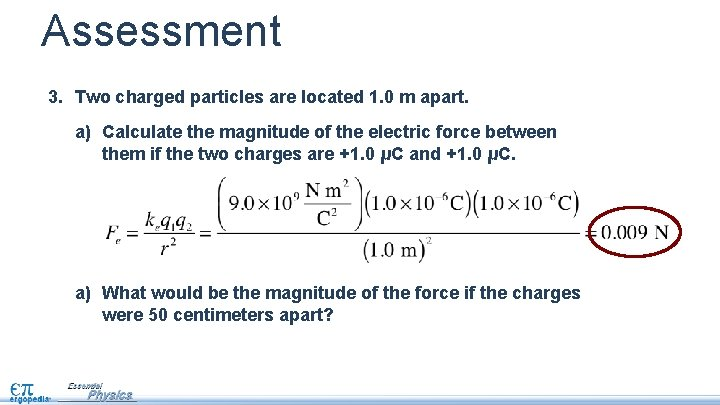Assessment 3. Two charged particles are located 1. 0 m apart. a) Calculate the magnitude of the electric force between them if the two charges are +1. 0 μC and +1. 0 μC. a) What would be the magnitude of the force if the charges were 50 centimeters apart?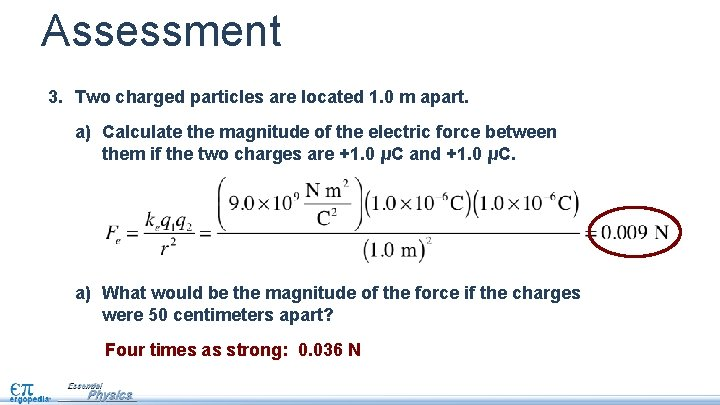Assessment 3. Two charged particles are located 1. 0 m apart. a) Calculate the magnitude of the electric force between them if the two charges are +1. 0 μC and +1. 0 μC. a) What would be the magnitude of the force if the charges were 50 centimeters apart? Four times as strong: 0. 036 N